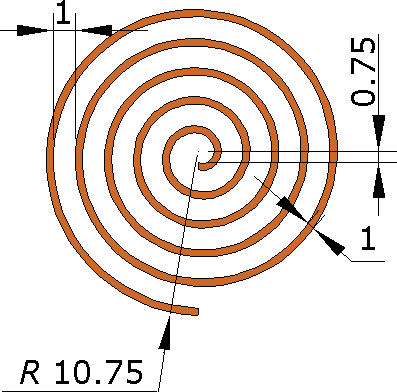QuickField

A new approach to field modelling
 Language: Global English Deutch Espanol Francais Italiano Danmark Ceske Chinese Pycckuü

>> >>

# Flat spiral coil inductance

This is an example of the flat (spiral) coil inductance calculation, performed with QuickField simulation software.

Problem type:
Axisymmetric problem of DC magnetics.

Geometry:All dimensions are in millimeters.

Given:
The wire diameter = 1 mm.
The wire material - copper;
The current in the coil I = 1 A.

Determine the inductance of the spiral coil.

Solution:
We represent the spiral coil in the form of concentric closed circles having the radii of the midlines of each turn. In this formulation, the problem can be modeled as an axisymmetric 2D.Inductance can be calculated from the energy of the magnetic field W:
L = 2*W/I2.

Results:
Flat concentric coil magnetic field distribution:The coil inductance L = 2*9.89*10-8 / 12 = 0.198 μH.

• Download simulation files (files may be viewed using any QuickField Edition).